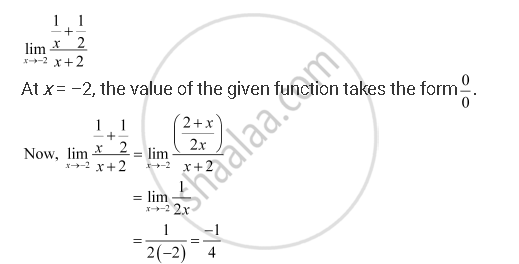CBSE (Commerce) Class 11CBSE
Share
Notifications

View all notifications
Books Shortlist
Your shortlist is empty

# Solution for Evaluate the Given Limit: Lim_(X -> -2) (1/X + 1/2)/(X + 2) - CBSE (Commerce) Class 11 - Mathematics

Login
Create free account

Forgot password?
ConceptIntuitive Idea of Derivatives

#### Question

Evaluate the Given limit: lim_(x -> -2) (1/x + 1/2)/(x + 2)

#### SolutionIs there an error in this question or solution?

#### APPEARS IN

NCERT Solution for Mathematics Textbook for Class 11 (2013 to Current)
Chapter 13: Limits and Derivatives
Q: 12 | Page no. 301
Solution Evaluate the Given Limit: Lim_(X -> -2) (1/X + 1/2)/(X + 2) Concept: Intuitive Idea of Derivatives.
S